SEARCH HOMEMath Central Quandaries & QueriesSubject: Piecewise Functions Name: Steph Who are you: Student I don't understand how to work this problem and do the graph for it. h(x)={-2 if x<0 {x+1 if 0< x <10 {-1/2x+16 if x>10 PLEASE HELPHi Steph,

I am going to look at a slightly different function.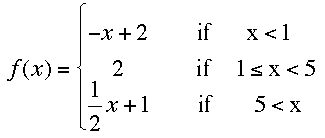This piecewise function is made from three different functions and I would start by graphing each individually.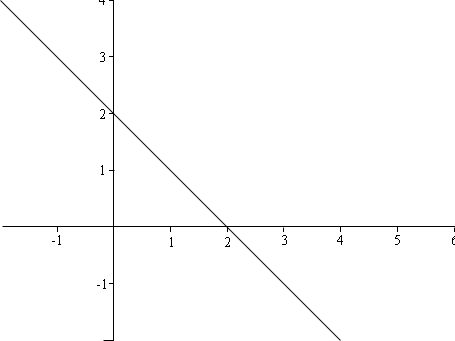y = -x + 2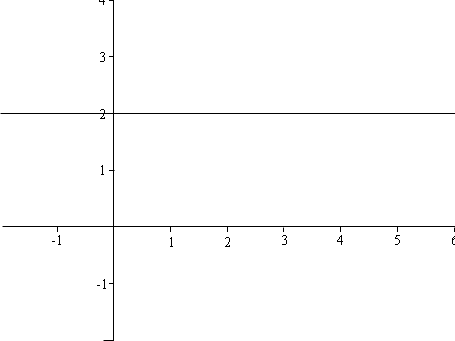y = 2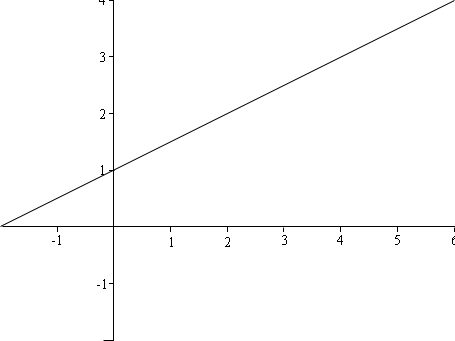y = 1/2 x + 1

You only want the piece of the first graph in the region of the palne described by x < 1.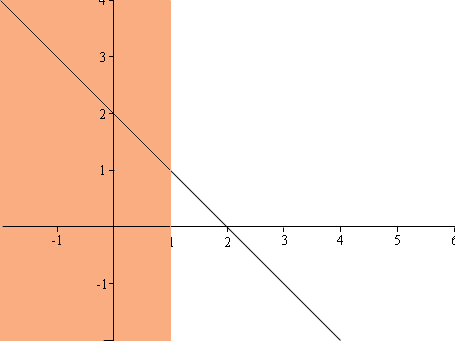You only want the piece of the second graph in the region of the palne described by 1 ≤ x < 5 .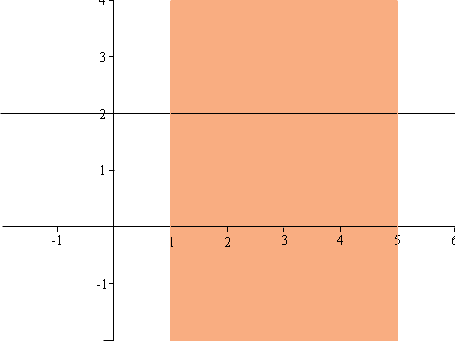You only want the piece of the third graph in the region of the palne described by 5 < x.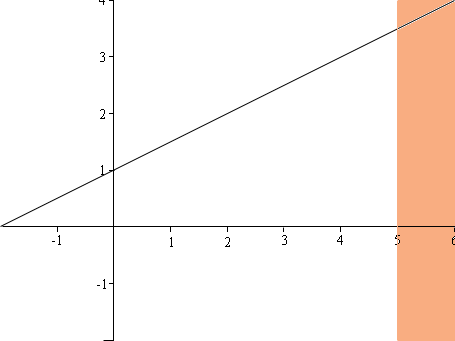I then cut out these three pieces and put them om one graph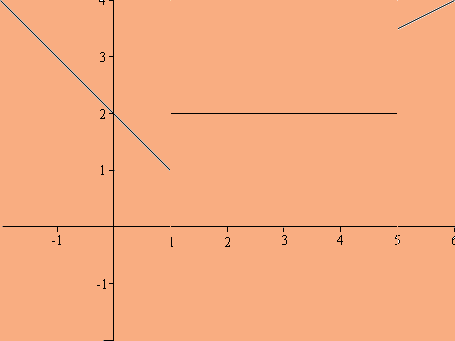I'm almost done. The problem I see is that the algebraic description of the function says that when x = 1 the value of the function is 2, and this is not clear from the graph. I would put at dot at (1,2) to show this.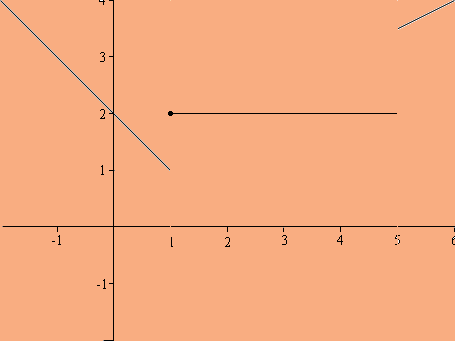graph of y = f(x)

PennyMath Central is supported by the University of Regina and The Pacific Institute for the Mathematical Sciences.